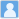• ### Determination of neutrino mass ordering in future $^76$Ge-based neutrinoless double-beta decay experiments

分类： 物理学 >> 核物理学 提交时间： 2016-09-14Jue Zhang Shun Zhou

摘要：Motivated by recent intensive experimental efforts on searching for neutrinoless double-beta decays, we perform a detailed analysis of the physics potential of the experiments based on 76Ge. Assuming no signals, current and future experiments could place a 90% lower limit on the half life T0ν1/2≳4×1026 yr and T0ν1/2≳7×1027 yr, respectively. Then, how to report an evidence for neutrinoless double-beta decays is addressed by following the Bayesian statistical approach. For the first time, we present a quantitative description of experimental power to distinguish between normal and inverted neutrino mass orderings. Taking an exposure of 104 kg⋅yr and a background rate of 10−4 counts/(keV⋅kg⋅yr), we find that a moderate evidence for normal neutrino mass ordering (i.e., with a Bayes factor B given by ln(B)≃2.5 or a probability about 92.3% according to the Jeffreys scale) can be achieved if the true value of effective neutrino mass mββ turns out to be below 0.01 eV.

同行评议状态:待评议